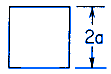Related Resources: calculators

### Solid Square Section Torsional Deformation and Stress Torsional Deformation Stress Equation and Calculator

Beam Deflection and Stress Equation and Calculators

Torsional Deformation and Stress Solid Square Section Equations and CalculatorALL calculators require a Premium Membership

θ = T L / K G

K = 2.25 a4

τmax = 0.601 T / a3 at Midpoint of each side

Where:

θ - angle of twist (radians)
T = Twisting or torque moment force-length, (in-lbs, N-mm)
L = Length (in, mm)
a = Distance as indicated (in, mm)
τ = Unit shear stress force / area (lbs/in2, N/mm2)
G = Modulus of rigidity force / area (lbs/in2, N/mm2)
K = Polar Moment of Inertia (in4, mm4) for section© Copyright 2000 - 2019, by Engineers Edge, LLC www.engineersedge.com